# If-then-else branching and logical tools

If-then-else logic is a concept for performing actions based on different conditions. If-then-else logic can be explained as follows: if the condition is true, then perform an action, else perform a different action.

In ModelBuilder, if-then-else logic can be added using the set of built-in logical tools or with custom Python functions or script tools.

## Logical tools

Logical tools are a category of ModelBuilder utilities that help you control the flow of processes in a model and enable if-then-else branching logic.

UtilityDescription

If Data Exists

Evaluates if the specified data exists.

If Field Exists

Evaluates if the input data has the specified fields.

If Selection Exists

Evaluates if the input data has a selection and if a certain number of records are selected.

If Coordinate System Is

Evaluates the input data for the specified coordinate system.

If Data Type Is

Evaluates if the input data matches the specified data type.

If Feature Type Is

Evaluates if a feature class is of the specified feature type.

If Field Value Is

Evaluates if the values in an attribute field match a specified value, expression, or second field.

If Row Count Is

Evaluates the row count of the input data and checks whether it matches a specified value.

If Spatial Relationship Is

Evaluates whether the inputs have a specified spatial relationship.

If Value Is

Evaluates an input value compared to a single value, a list of values, or a range of values using a defined comparison operator.

Merge Branch

The Merge Branch tool merges two or more logical branches into a single output.

Stop

Stops iteration if all input values meet the specified condition of true or false. It is functionally similar to the While iterator but is useful to stop a model if there is one While iterator in a model and no additional iterators can be added.

## Python

In addition to the built-in logical tools in ModelBuilder, you can write your own functions and tools to perform if-then-else branching using custom Python functions with the Calculate Value tool. These functions can test conditions using a variety of arcpy and other Python capabilities, and output Boolean true and false variables. Similarly, you can write a Python script that tests conditions and outputs Boolean true and false variables, make the script a geoprocessing script tool, and add the script tool to your model.

## Understanding conditions

Before choosing from the system tools or writing custom scripts, it is good practice to understand the conditional logic based on vector or raster data types.

### Run a model only if a file exists

The following example uses the If Data Exists tool to check for the existence of data in a particular workspace, and if data does exist, continue running the next tool, Add Field, in the model. If it does not exist, copy the feature class from a backup location before adding a field.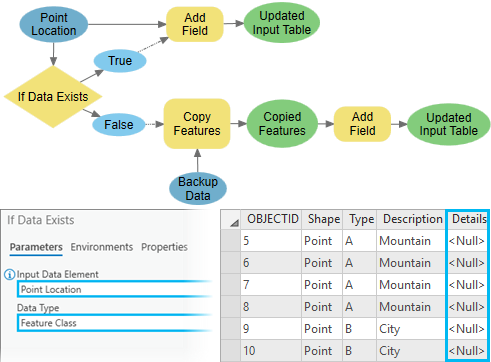### Run a model only if an input has a specific coordinate system

The following example uses the If Coordinate System Is tool to check whether the Coordinate System value of the input feature class is GCS_WGS_1984. If the Boolean output is False, the model will use the Project tool to project the input feature class to GCS_WGS_1984.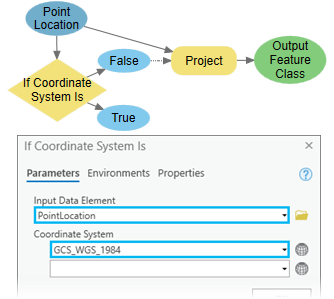### Run a model only if an input feature class is a particular geometry type

The following example uses the If Feature Type Is tool to check whether the input's feature type is Point. If the Boolean output is True, run the next tool, Add Locations. If the Boolean output is False, convert the input Feature To Point tool before running the Add Location tool. The Merge Branch tool merges the True and False branches so that the Solve tool can be run on either branch.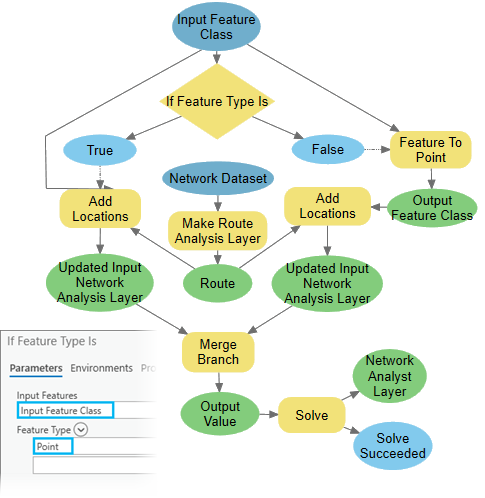### Modify field values based on a condition

The following example uses the If Field Value Is tool to check whether the input feature class has any records with the Type field value equal to A. If the Boolean output is True, select type A features using the Select Layer By Attribute tool, and modify the Description field values using the Calculate Field tool.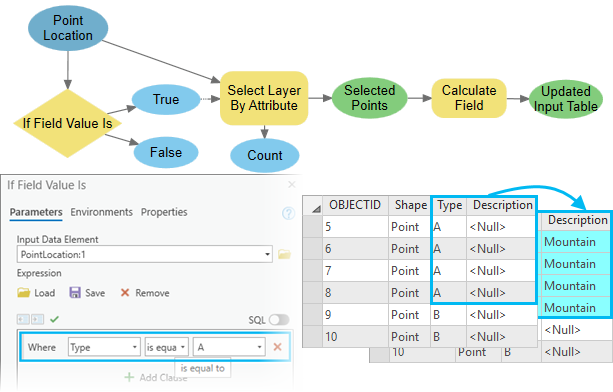### Run a model only if an SQL query results in any selected features

The following example uses the If Selection Exists tool to check whether the input feature class has any selected features. If the Boolean output is True, use the Calculate Field tool to calculate values of the Description field. If the Boolean output is False, use the Select Layer By Attribute tool to make the selection and modify field values.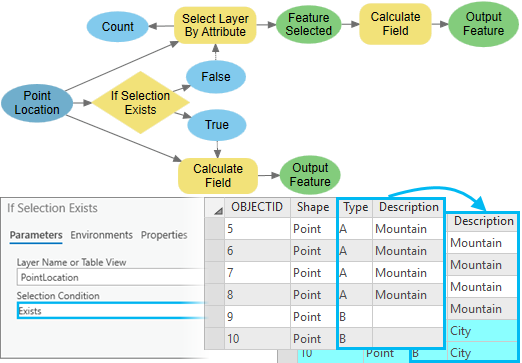### Run a model only if an input is a specific data type

The following example uses the Parse Path tool to get the file extension. The If Value Is tool checks whether the extension matches the Values parameter. The model only copies raster data with a .tif extension.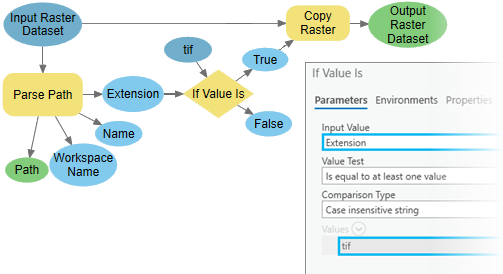### Perform a Boolean evaluation of a raster

The Test, Con, Greater Than, and Raster Calculator tools can be used to perform a Boolean evaluation of raster. In the following example, if a cell value is greater than 2000, then set to 1 (True), else set to 0 (False).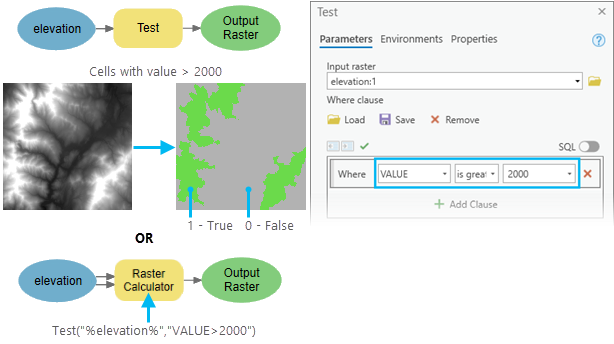### Perform a conditional evaluation of a raster

The Con or Raster Calculator tool can be used to conditionally evaluate a raster. In the following example, if a cell value is greater than 2000, then keep the input value, else set to NoData. Leaving the Con tool Input false raster or constant value parameter empty sets the value to NoData. Optionally, you can provide another raster or a constant value for the Input false raster or constant value parameter.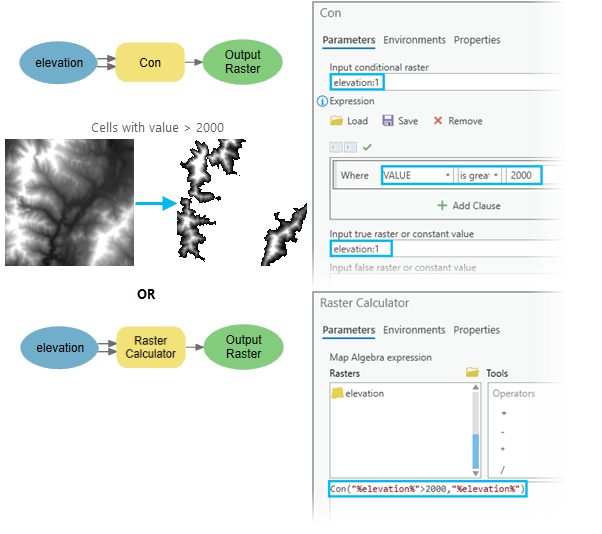### Extract cells from a raster with a query

The Extract By Attribute, Make Raster Layer, and Select Layer By Attribute tools can be used to extract cells from a raster. The following example shows methods to create a raster or a raster layer with cell values greater than 2000.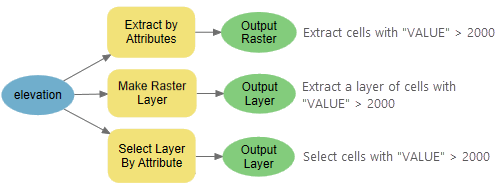### Extract cells from a raster with a query and set them to NoData

The Set Null, Reclassify, and Raster Calculator tools can be used to extract cells from a raster and set them to NoData. If the cell value is greater than 2000, then set to NoData, else use the input data value.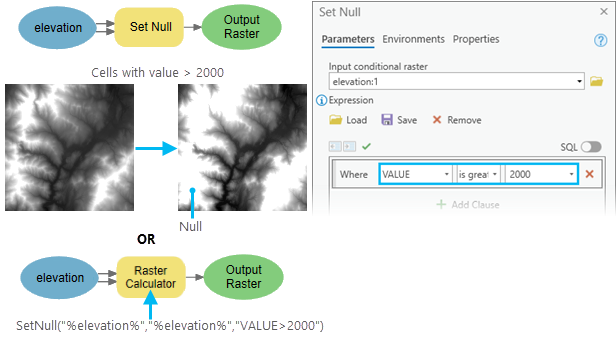### Extract NoData cells from a raster with a query and modify them

The Is Null tool with the Con tool, or the Raster Calculator tool can be used to extract NoData cells from a raster and modify them. In the following example, if a cell value is NoData, then set to 0, else use the input data value.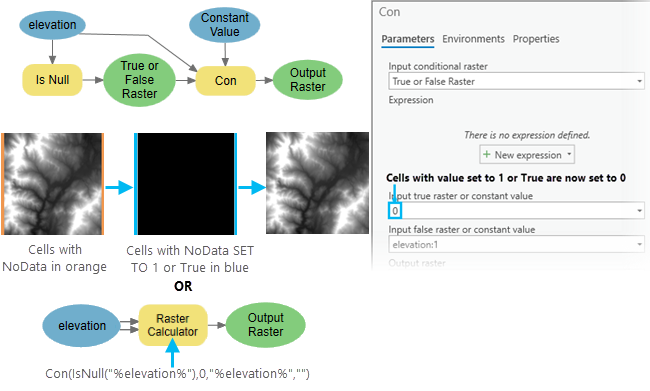### Run a model only if a raster property matches a specific condition

For example, to check whether the mean value of all cells is greater than 100, you can use the Get Raster Properties and If Value Is tools.

### Continue or stop a tool from running in a model based on a condition

The While and Stop tools can be used to continue or stop a model based on a condition.